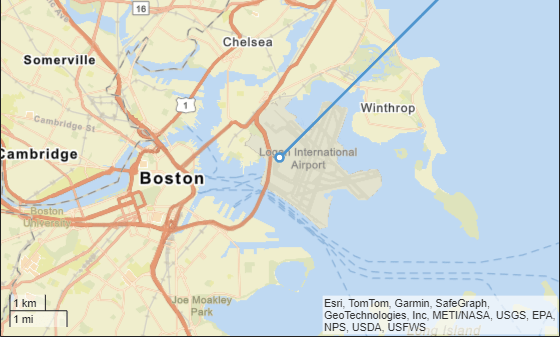# local2latlon

Convert local Cartesian coordinates to geographic coordinates

## Syntax

``[lat,lon,alt] = local2latlon(xEast,yNorth,zUp,origin)``

## Description

example

````[lat,lon,alt] = local2latlon(xEast,yNorth,zUp,origin)` converts point locations given by `xEast`, `yNorth`, and `zUp` from local Cartesian coordinates to geographic coordinates returned as `lat`, `lon`, and `alt`. `origin` specifies the anchor of the local coordinate system as a three-element vector of the form [latOrigin,lonOrigin,altOrigin]. Local coordinates `xEast`, `yNorth`, and `zUp` align with the east, north, and up directions, respectively. `alt` and altOrigin are altitudes as typically returned by GPS sensors.```

## Examples

collapse all

Establish an anchor point in the geographic coordinate system. These latitude and longitude coordinates specify Boston, MA.

`origin = [42.3648, -71.0214, 10.0];`

Generate local route in Cartesian coordinates, x, y, and z.

```z = zeros(1,101); % maintain height of 0 m x = 0:1000:100000; % 100 km in 1 km increments y = x; % move 100 km northeast```

Convert the local route coordinates to geographic coordinates, latitude and longitude.

`[lat,lon] = local2latlon(x,y,z,origin);`

Visualize the route on a map.

```zoomLevel = 12; player = geoplayer(lat(1),lon(1),zoomLevel); plotRoute(player,lat,lon);```## Input Arguments

collapse all

x-coordinates in the local Cartesian coordinate system, specified as a numeric scalar or vector, in meters.

Example: `-2.7119`

Data Types: `single` | `double`

y-coordinates in the local Cartesian coordinate system, specified as a numeric scalar or vector, in meters.

Example: `-7.0681`

Data Types: `single` | `double`

y coordinate in local Cartesian coordinate system, specified as a numeric scalar or vector, in meters.

Example: `-0.2569`

Data Types: `single` | `double`

Anchor of local coordinate system, specified as a three-element vector of the form [latOrigin,lonOrigin,altOrigin].

Example: `[42.3648 -71.0214 10]`

Data Types: `single` | `double`

## Output Arguments

collapse all

Latitude, in degrees, returned as a numeric scalar or vector.

`lat` is the same class as `xEast`. However, if any of the input arguments is of class `single`, then `lat` is of class `single`.

Longitude, in degrees, returned as a numeric scalar or vector.

`lon` is the same class as `yNorth`. However, if any of the input arguments is of class `single`, then `lon` is of class `single`.

Altitude, in meters, returned as a numeric scalar or vector, the same class as `zUp`.

`alt` is the same class as `zUp`. However, if any of the input arguments is of class `single`, then `alt` is of class `single`.

## Tips

• The latitude and longitude of the geographic coordinate system use the WGS84 standard that is commonly used by GPS receivers.

• This function defines altitude as the height, in meters, above the WGS84 reference ellipsoid.

• Some GPS receivers use standards other than WGS84. Conversions using other ellipsoids are available in the Mapping Toolbox. This function addresses the most common conversion between geographic locations and Cartesian coordinates used by the on-board sensors of a vehicle.

## Version History

Introduced in R2020a